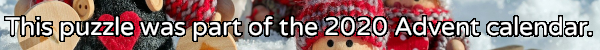mscroggs.co.uk
mscroggs.co.uksubscribe

# Puzzles

## 15 DecemberWhen talking to someone about this Advent calendar, you told them that the combination of XMAS and MATHS is GREAT. They were American, so asked you if the combination of XMAS and MATH is great; you said SURE. You asked them their name; they said SAM.
Each of the letters E, X, M, A, T, H, S, R, U, and G stands for a different digit 0 to 9. The following sums are correct:
Today's number is SAM. To help you get started, the letter T represents 4.

## 15 DecemberThere are 5 ways to make 30 by multiplying positive integers (including the trivial way):
• 30
• 2×15
• 3×10
• 5×6
• 2×3×5
Today's number is the number of ways of making 30030 by multiplying.

## Not Roman numerals

The letters $$I$$, $$V$$ and $$X$$ each represent a different digit from 1 to 9. If
$$VI\times X=VVV,$$
what are $$I$$, $$V$$ and $$X$$?

## 20 DecemberToday's number is the sum of all the numbers less than 40 that are not factors of 40.

## 15 DecemberToday's number is smallest three digit palindrome whose digits are all non-zero, and that is not divisible by any of its digits.

## 13 DecemberThere is a row of 1000 lockers numbered from 1 to 1000. Locker 1 is closed and locked and the rest are open.
A queue of people each do the following (until all the lockers are closed):
• Close and lock the lowest numbered locker with an open door.
• Walk along the rest of the queue of lockers and change the state (open them if they're closed and close them if they're open) of all the lockers that are multiples of the locker they locked.
Today's number is the number of lockers that are locked at the end of the process.
Note: closed and locked are different states.

## 7 DecemberThere is a row of 1000 closed lockers numbered from 1 to 1000 (inclusive). Near the lockers, there is a bucket containing the numbers 1 to 1000 (inclusive) written on scraps of paper.
1000 people then each do the following:
• Pick a number from the bucket (and don't put it back).
• Walk along the row of lockers and change the state (open them if they're closed and close them if they're open) of all the lockers that are multiples of the number they picked (including the number they picked).
Today's number is the number of lockers that will be closed at the end of this process.

## 6 DecemberThis puzzle is inspired by a puzzle that Daniel Griller showed me.
Write down the numbers from 12 to 22 (including 12 and 22). Under each number, write down its largest odd factor*.
Today's number is the sum of all these odd factors.
* If a number is odd, then its largest odd factor is the number itself.

## Archive

Show me a random puzzle
▼ show ▼